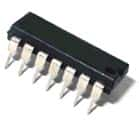# NTE2321 Quad General Purpose NPN Transistor NTEManufacturer: NTE
Part Number: NTE2321

Price: \$4.62
Sorry, we are currently sold out of
'NTE2321 Quad General Purpose NPN Transistor NTE'.
Code: IC433PD12
Volume Pricing
Quantity Price Each
1-9\$4.62
10+\$4.30
Quantity:

Silicon NPN
14 Pin Dip Package

Electrical Characteristics (TA=25�C unless otherwise noted):

OFF
Collector�Emitter Breakdown Voltage: 40V min.
- (IC = 10mA, IB = 0)
Collector�Base Breakdown Voltage: 60V min.
- (IC = 10uA, IE = 0)
Emitter�Base Breakdown Voltage: 5V min.
- (IE = 10uA, IC = 0)
Collector Cutoff Current: 50nA max.
- (VCB = 50V, IE = 0)
Emitter Cutoff Current: 50nA
- (VEB = 3V, IE = 0)

ON
DC Current Gain: 100 min.
- (VCE = 10V, IC = 150mA)
Collector�Emitter Saturation Voltage: 0.4V
- (IC = 150mA, IB = 15mA)

SMALL SIGNAL
Current Gain�Bandwidth Product: 200MHz min.
- (VCE = 20V, IC = 20mA, f = 100MHz)
Output Capacitance: 8pF max.
- (VBE = 19V, IE = 0, f = 1MHz)
Input Capacitance: 30pF max.
- (VBE = 0.5V, IC = 0, f = 1MHz)

Manufactured by: NTE
Part Number: NTE2321

Full Datasheet: NTE2321